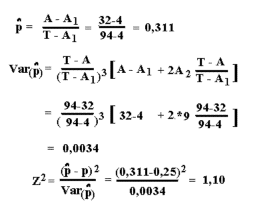### 5.7 Calculation of corrected segregation ratio according to Singles method

The method is to be used when your data are collected in the field, and you only find useful families where at least one affected offspring occur.

 Set digit for print ->

Put your data in the second line and press the Calculate button.

Example:
The disease tyrosinemia in mink is investigated by the following set of field data for recessive Mendelian inheritance. A description of the disease tyrosinemia in mink are published by Christensen et al. Canadian J. Comparative Medicine, 43:333-340, 1979.

In the data set 16 litters are included with at least one affected offspring. The observed numbers are given below.

```T = 94   - total numbers of pups
A = 32   - total number of affected
A1 = 4   - number of families with one affected pup
A2 = 9   - number of families with two affected pups```
The applied formulas for test of Mendelian inheritance by "Singles method" (with the proportion p-hat ) are shown in the figure below. The tyrosinemia data are applied to show the use of the formulas. The results below are rounded, so they are sligthly different from what you get using the above calculator.The Z2 are Chi-squared distributed with 1 degree of freedom. The test of p-hat is against 0,25, this is the expected ratio when both parents are carrier and there is recessive inheritance. The test value is less than 3.84. Therefore the deviations can be accepted as not statistically significant.

Questions:
Calculate the corrected segregation ratio for the following observation set of field data, where there has been at least one affected per family.
```T =  30  - total numbers of observations
A =  16  - total number of affected
A1 = 12  - number of families with one affected
A2 =  2  - number of families with two affected ```

Is the segregation ratio statistically significant different from 1:3 segregation.

Back to theory, Back to theory (in Danish) or Back to the other programs